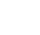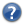CatalogLooking for catalogs ..J/AJ/148/54 1114 The Hypatia Catalog ((Hinkel+, 2014) ReadMe+ftp2014AJ....148...54HSimilar Catalogs 1. J/AJ/148/54/rhypatia Reduced Hypatia Catalog of stellar abundances (2685 rows)

Query by Constraintsapplied on Columns (Output Order: + - )
ShowSortColumn  Constraint Explain   (UCD)
HIP  (i) Hipparcos number
Fe/H [Sun] (n) Abundance [Fe/H] (Z=26)
Li/Fe [Sun] (n) Abundance [Li/Fe] (Z=3)
Be/Fe [Sun] (n) Abundance [Be/Fe] (Z=4)
C/Fe [Sun] (n) Abundance [C/Fe] (Z=6)
N/Fe [Sun] (n) Abundance [N/Fe] (Z=7)
O/Fe [Sun] (n) Abundance [O/Fe] (Z=8)
Na/Fe [Sun] (n) Abundance [Na/Fe] (Z=11)
Mg/Fe [Sun] (n) Abundance [Mg/Fe] (Z=12)
Al/Fe [Sun] (n) Abundance [Al/Fe] (Z=13)
Si/Fe [Sun] (n) Abundance [Si/Fe] (Z=14)

ALL cols

 (n) indicates a possible blank or NULL column (i)indexed column
P/Fe [Sun] (n) Abundance [P/Fe] (Z=15)
S/Fe [Sun] (n) Abundance [S/Fe] (Z=16)
K/Fe [Sun] (n) Abundance [K/Fe] (Z=19)
Ca/Fe [Sun] (n) Abundance [Ca/Fe] (Z=20)
CaII/Fe [Sun] (n) Abundance [CaII/Fe] (Z=20)
Sc/Fe [Sun] (n) Abundance [Sc/Fe] (Z=21)
ScII/Fe [Sun] (n) Abundance [ScII/Fe] (Z=21)
Ti/Fe [Sun] (n) Abundance [Ti/Fe] (Z=22)
TiII/Fe [Sun] (n) Abundance [TiII/Fe] (Z=22)
V/Fe [Sun] (n) Abundance [V/Fe] (Z=23)
VII/Fe [Sun] (n) Abundance [VII/Fe] (Z=23)
Cr/Fe [Sun] (n) Abundance [Cr/Fe] (Z=24)
CrII/Fe [Sun] (n) Abundance [CrII/Fe] (Z=24)

ALL cols

 (n) indicates a possible blank or NULL column (i)indexed column
Mn/Fe [Sun] (n) Abundance [Mn/Fe] (Z=25)
Co/Fe [Sun] (n) Abundance [Co/Fe] (Z=27)
Ni/Fe [Sun] (n) Abundance [Ni/Fe] (Z=28)
Cu/Fe [Sun] (n) Abundance [Cu/Fe] (Z=29)
Zn/Fe [Sun] (n) Abundance [Zn/Fe] (Z=30)
Sr/Fe [Sun] (n) Abundance [Sr/Fe] (Z=38)
SrII/Fe [Sun] (n) Abundance [SrII/Fe] (Z=38)
Y/Fe [Sun] (n) Abundance [Y/Fe] (Z=39)
YII/Fe [Sun] (n) Abundance [YII/Fe] (Z=39)
Zr/Fe [Sun] (n) Abundance [Zr/Fe] (Z=40)
ZrII/Fe [Sun] (n) Abundance [ZrII/Fe] (Z=40)
Mo/Fe [Sun] (n) Abundance [Mo/Fe] (Z=42)
Ru/Fe [Sun] (n) Abundance [Ru/Fe] (Z=44)

ALL cols

 (n) indicates a possible blank or NULL column (i)indexed column
BaII/Fe [Sun] (n) Abundance [BaII/Fe] (Z=56)
La/Fe [Sun] (n) Abundance [La/Fe] (Z=57)
LaII/Fe [Sun] (n) Abundance [LaII/Fe] (Z=57)
Ce/Fe [Sun] (n) Abundance [Ce/Fe] (Z=58)
CeII/Fe [Sun] (n) Abundance [CeII/Fe] (Z=58)
Pr/Fe [Sun] (n) Abundance [Pr/Fe] (Z=59)
Nd/Fe [Sun] (n) Abundance [Nd/Fe] (Z=60)
Sm/Fe [Sun] (n) Abundance [Sm/Fe] (Z=62)
Eu/Fe [Sun] (n) Abundance [Eu/Fe] (Z=63)
EuII/Fe [Sun] (n) Abundance [EuII/Fe] (Z=63)
Gd/Fe [Sun] (n) Abundance [Gd/Fe] (Z=64)
Dy/Fe [Sun] (n) Abundance [Dy/Fe] (Z=66)
Hf/Fe [Sun] (n) Abundance [Hf/Fe] (Z=72)
Pb/Fe [Sun] (n) Abundance [Pb/Fe] (Z=82)
 Adapt form Display your selection only Reset to default columnsDisplay UCD1+    UCD1